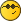A question on Pendulum experimentjmnkJunior MemberPosts: 13 Threads: 2 Joined: Nov 2020 12-26-2020, 03:48 AM (12-24-2020, 03:56 AM)solid Wrote: x = A sin(omega t)                                      # deviation angle d sin(omega t) /dt= omega cos(omega t) v = dx/dt = (A omega) cos(omega t)          # rotation speed s = dA²omega²                                           # square standard deviation 'amplitude' calculated (dA² omega²)/omega/omega = dA² represents the standard deviation of the angle (for small harmonic oscillations)...i think. This example is too complicated for a tutorial... hmm, I think I'm not getting it. I'm perfectly following up to this: v = dx/dt = (A omega) cos(omega t)          # rotation speed but I do not understand this equation (or perhaps I'm misinterpreting the notation): s = dA²omega²  # square standard deviation the standard deviation of: (A omega) cos(omega t) is, I think, A*omega/√2, so the square standard deviation would be A²omega²/2.  What does 'd' in this equation represent: s = dA²omega²  ? Either way, the code at no point appears to be using the _square_ of standard deviation, so why is that stddev divided by (omega * omega) to get amplitude? If the code was  ' stddev * √2 / omega ' then I would understand why the result is 'amplitude'.  (Although I think it would need to be stddev * √2 / (2*pi*frequency) since in the code frequency is in 1/s rather then in rad/s) « Next Oldest | Next Newest »